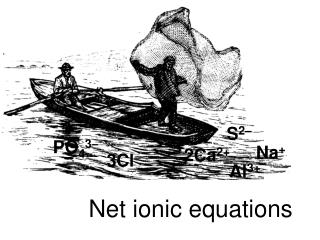DownloadDownload PresentationNet ionic equations

# Net ionic equations

Télécharger la présentation## Net ionic equations

- - - - - - - - - - - - - - - - - - - - - - - - - - - E N D - - - - - - - - - - - - - - - - - - - - - - - - - - -
##### Presentation Transcript

1. Net ionic equations S2– PO43– Na+ 2Ca2+ 3Cl– Al3+

2. Review: forming ions • Ionic (i.e. salt) refers to +ve ion plus -ve ion • Usually this is a metal + non-metal or metal + polyatomic ion (e.g. NaCl, NaClO3, Li2CO3) • Polyatomic ions are listed on page 95 • (aq) means aqueous (dissolved in water) • For salts (aq) means the salt exists as ions • NaCl(aq) is the same as: Na+(aq) + Cl–(aq) • Acids form ions: HCl(aq) is H+(aq) + Cl–(aq), Bases form ions: NaOH(aq) is Na+ + OH– Q - how is charge determined (+1, -1, +2, etc.)? A - via valences (periodic table or see pg. 95) • F, Cl gain one electron, thus forming F–, Cl– • Ca loses two electrons, thus forming Ca2+

3. Background: valences and formulas • Charge can also be found via the compound • E.g. in NaNO3(aq) if you know Na forms Na+, then NO3 must be NO3– (NaNO3 is neutral) • By knowing the valence of one element you can often determine the other valences Q - Write the ions that form from Al2(SO4)3(aq)? Step 1 - look at the formula: Al2(SO4)3(aq) Step 2 - determine valences: Al3 (SO4)2 • (Al is 3+ according to the periodic table) • Step 3 - write ions: 2Al3+(aq) + 3SO42–(aq) • Note that there are 2 aluminums because Al has a subscript of 2 in the original formula

4. Practice with writing ions Q - Write ions for Na2CO3(aq) A - 2Na+(aq) + CO32–(aq) (from the PT Na is 1+. There are 2, thus we have 2Na+. There is only one CO3. It must have a 2- charge) • Notice that when ions form from molecules, charge can be separated, but the total charge (and number of each atom) stays constant. Q - Write ions for Ca3(PO4)2(aq) & Cd(NO3)2(aq) A - 3Ca2+(aq) + 2PO43–(aq) A - Cd2+(aq) + 2NO3–(aq) Q - Write ions for Na2S(aq) and Mg3(BO3)2(aq) A - 2Na+(aq) + S2–(aq), 3Mg2+(aq)+ 2BO33–(aq)

5. Types of chemical equations Equations can be divided into 3 types (pg. 333) 1) Molecular, 2) Ionic, 3) Net ionic • Here is a typical molecular equation: Cd(NO3)2(aq) + Na2S(aq)  CdS(s) + 2NaNO3(aq) • We can write this as an ionic equation (all compounds that are (aq) are written as ions): Cd2+(aq) + 2NO3–(aq) + 2Na+(aq) + S2–(aq)  CdS(s) + 2Na+(aq) + 2NO3–(aq) • To get the NET ionic equation we cancel out all terms that appear on both sides: Net: Cd2+(aq) + S2–(aq)  CdS(s)

6. Equations must be balanced • There are two conditions for molecular, ionic, and net ionic equations Materials balance Both sides of an equation should have the same number of each type of atom Electrical balance Both sides of a reaction should have the same net charge Q- When NaOH(aq) and MgCl2(aq) are mixed, _______(s) and NaCl(aq) are produced. Write balanced molecular, ionic & net ionic equations Mg(OH)2

7. NaOH(aq) + MgCl2(aq)  Mg(OH)2(s) + NaCl(aq) Next, balance the equation 2 2 Ionic equation: 2Na+(aq) + 2OH-(aq) + Mg2+(aq) + 2Cl-(aq)  Mg(OH)2(s) + 2Na+(aq) + 2Cl-(aq) First write the skeleton equation Net ionic equation: 2OH-(aq) + Mg2+(aq)  Mg(OH)2(s) Write balanced ionic and net ionic equations: CuSO4(aq) + BaCl2(aq)  CuCl2(aq) + BaSO4(s) Fe(NO3)3(aq) + LiOH(aq)  ______(aq) + Fe(OH)3(s) Na3PO4(aq) + CaCl2(aq)  _________(s) + NaCl(aq) Na2S(aq) + AgC2H3O2(aq)  ________(aq) + Ag2S(s) LiNO3 Ca3(PO4)2 NaC2H3O2

8. Cu2+(aq) + SO42–(aq) + Ba2+(aq) + 2Cl–(aq)  Cu2+(aq) + 2Cl–(aq) + BaSO4(s) Net: SO42–(aq) + Ba2+(aq)  BaSO4(s) Fe3+(aq) + 3NO3–(aq) + 3Li+(aq) + 3OH–(aq)  3Li+(aq) + 3NO3–(aq) + Fe(OH)3(s) Net: Fe3+(aq) + 3OH–(aq)  Fe(OH)3(s) 2Na3PO4(aq) + 3CaCl2(aq) Ca3(PO4)2(s)+ 6NaCl(aq) 6Na+(aq) + 2PO43–(aq) + 3Ca2+(aq) + 6Cl–(aq)  Ca3(PO4)2(s)+ 6Na+(aq) + 6Cl–(aq) Net: 2PO43–(aq) + 3Ca2+(aq)  Ca3(PO4)2(s) 2Na+(aq) + S2–(aq) + 2Ag+(aq) + 2C2H3O2–(aq)  2Na+(aq) + 2C2H3O2–(aq) + Ag2S(s) Net: S2–(aq) + 2Ag+(aq)  Ag2S(s) For more lessons, visit www.chalkbored.com